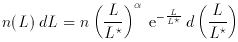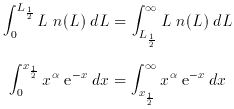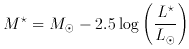### 2. OPTICAL LUMINOSITY FUNCTIONS

If we have a sample with distance information for every object, so that we know L(r, S) = 4r2 S for each galaxy, we can define a volume over which the sample is complete to any arbitrarily low luminosity, and compute the density of galaxies as a function of luminosity. This is the approach taken by Schechter (1976), using a sample from the Reference Catalog of Bright Galaxies (de Vaucouleurs and de Vaucouleurs 1967) which is magnitude limited at B0 = 11.75. An alternative method to derive n(L) is to study rich clusters which are distant enough that 1 / r2 is roughly constant for all members and rich enough that confusion due to foreground and background galaxies in the field is not a major problem (Oemler 1974). A compendium of various functional forms for n(L) is given by Felten (1977). The form chosen by Press and Schechter (1974) is very popular, not because it is simple, but because it follows from a theoretical analysis of self-similar gravitational condensation in the early universe. This is a three parameter function of the form:

7.where the parameters are n (the total density),(the power law slope for very low L), and L(the luminosity of the ``break'' where the slope of n(L) changes rapidly). For L greater than L, n(L) decreases exponentially. Typical values forlie in the range -1.5 << -1, so integrating over all luminosities the total number of galaxies diverges. This is not necessarily unphysical, since the total luminosity,L n(L) dL remains finite. Integrals of the Schechter function can often be expressed in terms of the incomplete gamma function (Davis 1964), e.g.,

8.An analog to the median luminosity galaxy is the ``half-light"'' object, for which half the total luminosity of the sample comes from galaxies of higher luminosity, half from lower:

9.which gives L1/2 = 0.16 L, i.e., about 2 magnitudes fainter than L. Expressing the luminosity parameter as a magnitude

10.we find Mtypically has a value in the range -23 < M+ 5 log h50 <-20 in the blue.

If the parametersand Lof the Schechter function were independent of position (i.e., a global form for the luminosity function varying only in its normalization, n), then many problems could be greatly simplified. For example, distances to clusters could be estimated by measuring the number of galaxies vs. apparent magnitude, and fitting L. (A more sophisticated treatment of this idea is given by Schechter and Press 1976.) The variation of the luminosity function among clusters has been studied by Dressler (1978) and more recently by Lugger (1986). Apparently there is variation of almost two magnitudes in Mfrom cluster to cluster, although this variation is not clearly associated with cluster properties; in particular the presence of a cD does not imply a depletion of moderate luminosity galaxies, supporting Merritt's (1985) conclusion that cD's are not growing significantly by cannibalism at present. It remains an open question whether either the parameters Land, or the form of the luminosity function itself, vary with large scale environment, e.g., between clusters and voids. This would be expected in a ``biased galaxy formation'' scenario (e.g., Dekel and Silk 1986) where the initial mass function of galaxies is strongly influenced by the local mass density.

Another question about the optical luminosity function which is currently discussed is whether different luminosity functions should be used for different galaxy types (Sandage et al. 1985, Binggeli 1987). If dwarf ellipticals and ordinary ellipticals are considered separately, the luminosity function of the larger galaxies is typically Gaussian; the same is true for irregulars and spirals. Virgo is the only cluster for which we have complete catalogs of galaxies faint enough to measure various luminosity functions for different galaxy types. It is known in many clusters that the relative abundance of different galaxy types is a strong function of the local density of galaxies (Dressler 1980), so it is not implausible that at least ellipticals and spirals might have different luminosity functions. This is certainly true at the very high end, where the (exclusively elliptical) cD's are often so far above Lthat their abundance is not well fit by the exponential cutoff of the Schechter function at the high end.

Assuming that different galaxy types have distinct luminosity functions, morphological segregation (e.g., Giovanelli and Haynes 1985, Dressler 1980) appears to require that the overall galaxy luminosity function vary with position, since varying the relative fractions of spiral, elliptical and S0 galaxies varies the contribution of each of their luminosity functions to the aggregate luminosity function. Since the colors of galaxies also correlate with type this means that the aggregate Schechter function parameters must also vary with color. Large scale structure in the galaxy distribution entails more than a variation in nwith position; in fact we may need a multivariate luminosity function, for example n(L,) whereis the density of galaxies in the immediate environment. Data which could be used to derive this function are presented by Haynes in this volume.